© 2021 - First Crazy Developer (Abhishek Kumar)

###Closures in JavaScript

When we talk about closure in JavaScript,it is a confusing topic as we compare this functionality to other language. Before going to understand the concept of closure as in single line I want to say it is gray area of JavaScript where function and variable scope intersect.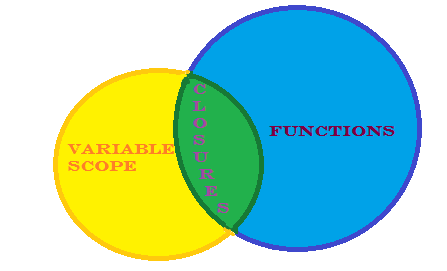In all other language when function returns all local variable not available or we can say not accessible. But when we talk about JavaScript  the variable of a function are kept alive, even after the function returns.

We will start from begining and then slowly move into hostile areas where closures can be found.

First we discuss concept of Functions within Functions:

Now we start to understand the concept  what happend when we use function within functions and return inner function from  parent function.

Go with the following code:

function  setName(firstName,lastName){

return firstName + ' ' + lastName;
}

var empName= setName('Abhishek','Kumar');
console.log(empName)

In the above code setName function take two arguments and return the value of those arguments where called it. In above example empName called the setName().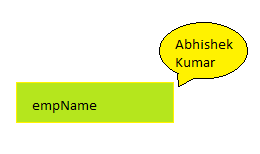So we know that JavaScript function can return anything. We can easily return different datatypes through function. In normal scenario when code that is calling the function knows what the function returns, we can easily handle that values.

But the twisting part is that in JavaScript we can even return another function within function.
Look the following code--

function  setName(firstName,lastName){
var companyName='Global Logic';
var empName=firstName + ' ' + lastName;

function  getName(){
}

return getName;
}

In above code there is function setName that contains alert and a child or inner function getName. So scenario is that there is an outer function and inner function.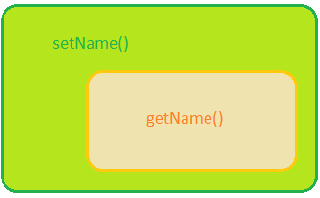Now what will be happen when we call parent function?

The twisting part is when we call setName function it returns the getName function:

Look the following code---

var tempVar=setName('Abhishek','Kumar');

When we run the above code, all of the code inside parent function will get execute. This means we have to alert dialog box with 'Parent Function' value.

After the completion of dialog box child function getName will be created and return to the variable tempVar. Now that variable have only reference of child function getname. We can say temVar only point to the child function. It has no any reference of parent function. Simply we can say parent function scope goes away. So now we can invoke child function through tempVar variable.

Look the following code---

var tempVar=setName('Abhishek','Kumar');
tempVar();

When we run the above code first parent function alert dialog box appeared and then inner function alert dialog box will be appeared.

The only thing that we may have newly found is realizing once a function returns a value, it is no longer around. The only thing that remains is the returned value.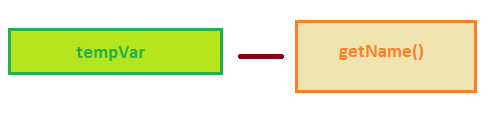Now we are getting closed to hostile territory.

In real scenario there are lots of variable defined which shared between the outer function and the inner function.
look the following code--

function totalHit(){

var totalCount=0;
var prefixVal='Total hits are=';

function countTotalHit(){
totalCount=totalCount+1;
}

return countTotalHit;
}

Above code in a simple way is to count total hit. So in above code there is parent function totalHit inside that function we have totalCount and prefixVal variable, we set 0 value in totalCount & some message in prefixVal.

we have also inner function named countTotalHit.

function countTotalHit(){
totalCount=totalCount+1;
}

In inner function we have totalCount variable, we add total count and set into totalCount and also displat alert dialog box with total count value. totalCount define earlier in parent function.

Now back to parent function totalHit, where in last line we return inner function named countTotalHit.

Now we look complete code which is similar as previous example, like we have a outer(parent) function inside that inner (child) function and we have the outer function returning the inner function.

Now look the following code --

var countHit=totalHit();
countHit() -->> in alert Total hits are=1
countHit() -->> in alert Total hits are=2
countHit() -->> in alert Total hits are=3

Above code we declare countHit variable and assign totalHit() into that. When we execute this code all code of  totalHit() executed and returned reference of inner function and assign to countHit variables.

As previously I said the outer function goes away when inner function return to the calling variable. What happens in the following line of code--

function countTotalHit(){
totalCount=totalCount+1;
}

In current inner function scope totalCount & prefixVal should be undefined but when we execute the above code the example obviously worked, so something else is going on here. That something else is the mysterious closure.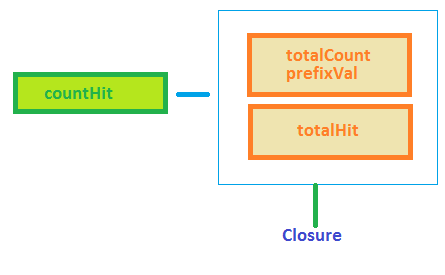The JavaScript runtime that keeps track of all of your variables, memory usage, references, and so on is really clever. In this example, it detects that the inner function (countTotalHit) is relying on some variables from the outer function (totalHit). When that happens, the runtime ensures that any variables in the outer function that are needed are still available to the inner function even if the outer function goes away.

So when parent function goes away before that all shared variables on which inner function relay are not destroyed. Instead that they are enclosed by the inner function the closure
.Home Page 07 June 2015